Definitions of Square Dance Calls and Concepts
Rotary Circulate [C4]

Index -->  Plus  |  A1  |  A2  |  C1  |  C2  |  C3A  |  C3B  |  C4  |  NOL  |
Definitions (Text Only) -->  Plus  |  A1  |  A2  |  C1  |  C2  |  C3A  |  C3B  |  C4  |  NOL  |
 Find call:

 \$B8@8l(B\$BJ?9T\$J(B Wave \$B\$+\$i(B.

Leaders \$B\$O(B, 1/2 Split Circulate, Circulate twice \$B\$r\$7(B, last half of a Split Circulate (Split Circulate \$B\$N8eH>(B) \$B\$r\$7\$^\$9(B. Trailers \$B\$O(B, Extend, Hinge, Box Circulate twice, Hinge \$B\$r\$7(B, Extend \$B\$r\$7\$^\$9(B.

\$BJ?9T\$J(B Wave \$B\$G=*\$o\$j\$^\$9(B.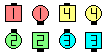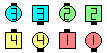Rotary Circulate\$B\$NA0(B \$B8e(B

\$B%@%s%9\$N%R%s%H(B: Trailers \$B\$N%Q!<%H\$O\$7\$P\$7\$P(B, Extend, Hinge, Box Counter Rotate 1/2 (\$BF1\$8?M\$H

\$BCm

• Rotary Circulate \$B\$O(B palindrome (\$B2sJ8(B, \$BA08e\$I\$A\$i\$+\$iFI\$s\$G\$bF10l\$KFI\$a\$k8l6g(B) \$B\$H\$J\$C\$F\$\$\$F(B, \$B%Q!<%H\$rA0\$+\$i9T\$C\$F\$b8e\$m\$+\$i9T\$C\$F\$b(B, \$BF1\$8F0\$-\$K\$J\$C\$F\$\$\$^\$9(B.
• \$BCm0U\$7\$F\$/\$@\$5\$\$(B: Rotary Motivate \$B\$^\$?\$O(B Percolate, Perk Up, Couple Up \$B\$J\$I(B) \$B\$G\$O(B, Rotary Circulate \$B\$r\$7\$F;X<(\$5\$l\$?%3!<%k\$r(B Finish \$B\$7\$^\$9(B. Rotary Spin \$B\$N%P%j%(!<%7%g%s\$N(B (Rotary anything) \$B\$G\$O\$"\$j\$^\$;\$s(B. \$B\$"\$k%3!<%i!<\$O(B, \$B\$"\$\$\$^\$\$\$5\$rHr\$1\$k\$?\$a\$K(B, Rotary Circulaters Motivate \$B\$H8@\$\$\$^\$9(B.
• \$B1~MQ(B: \$B1~MQ(B: \$B%3!<%i!<\$O(B, Ends \$B\$*\$h\$S(B Centers \$B\$N(B Circulate \$B\$GF0\$/%]%8%7%g%s\$N?t\$r(B, Rotary Circulate But Centers Circulate 3 spots \$B\$N\$h\$&\$K;X<(\$9\$k\$3\$H\$,\$G\$-\$^\$9(B.

Cheat: Follow Thru; Circulate twice; Any Shoulder 1/2 Tag.

Contour The Line [C4]: Contour The Line [C4]: \$B0lHLE*\$J(B Line\$B\$+\$i(B. Hinge; Box Circulate twice; Hinge \$B\$r\$7\$^\$9(B. Wave \$B\$^\$?\$O(B Inverted Line \$B\$G=*\$o\$j\$^\$9(B. 4 \$B%Q!<%H\$N%3!<%k\$G\$9(B.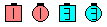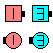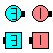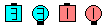Contour The Line\$B\$NA0(B Hinge\$B\$N8e(B Box Circulate twice\$B\$N8e(B Hinge\$B\$N8e(B (\$B=*\$o\$j(B)Comments? Questions? Suggestions?